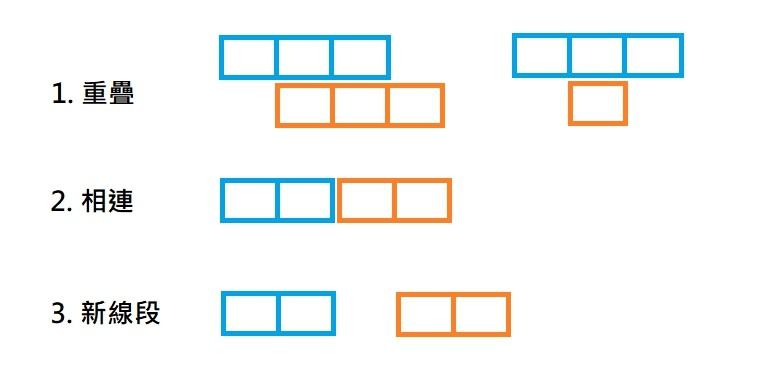#0

## [C++][APCS] 線段覆蓋長度

``````#include <stdio.h>
#include <stdlib.h>
#include <cmath>
#include <set>

using namespace std;

#define MAXM 1e8

//線段類別
class Line
{
public:
int start; //線段開始位置
int end;   //線段結束位置

public:
Line(int start, int end)
{
this->start = start;
this->end = end;
}
};

//線段的比較方式
struct LineCompare
{
bool operator()(const Line *a, const Line *b)
{
return a->start < b->start;
}
};

int main(void)
{
int n, s, e;
scanf("%d", &n);

multiset<Line *, LineCompare> lines;

for (int i = 0; i < n; i++)
{
scanf("%d %d", &s, &e);
lines.insert(new Line(s, e));
}
//多新增一段長度為0的線段放在最後
lines.insert(new Line(MAXM, MAXM));

int count = 0;

auto it = lines.begin();
int start = (*it)->start;
int end = (*it)->end;
it++;

for (it = it; it != lines.end(); it++)
{
//線段重疊或相連
if ((*it)->start <= end)
{
end = max(end, (*it)->end);
}
//新線段
else
{
//計算當前線段長度
count += end - start;
start = (*it)->start;
end = (*it)->end;
}
}

//印出解答
printf("%d\n", count);

//釋放記憶體
for (it = lines.begin(); it != lines.end(); it++)
{
delete *it;
}

system("pause");
return 0;
}
``````

``````4
5 6
1 2
4 8
7 9
``````
``````5
160 180
150 200
280 300
300 330
190 210
``````
``````1
120 120
``````

``````6
``````
``````110
``````
``````0
````````````end = max(end, (*it)->end);
``````

``````lines.insert(new Line(MAXM, MAXM));
``````